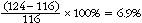1332.0.55.002 - Statistical Language!, 2008
ARCHIVED ISSUE Released at 11:30 AM (CANBERRA TIME) 27/06/2008  First Issue
Page tools:Print All
Contents >> Index >> How can we calculate an Index?

HOW CAN WE CALCULATE AN INDEX?

Indexes are calculated by comparing variations in quantity from one time period to the next. For a price index the calculation is the price from one time period divided by the base period price. In the following example the 'Widget Price Index" started in 2005. The following table demonstrates how a 'Widget Price Index' would be calculated in 2006 and 2007. The percentage change in the price index from 2006 to 2007 isPrices of Widgets 2005-2007Year Price per Widget (\$) Widget Price Index2005 50 1002006 58 58/50x100=1162007 62 62/50x100=124Previous Page Next Page